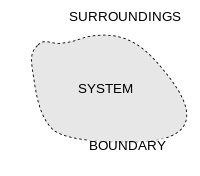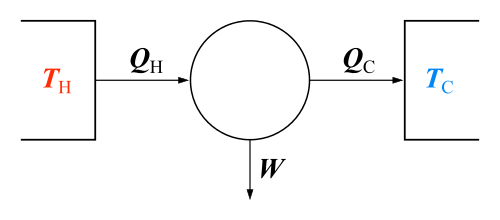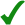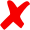Thermodynamic system

A thermodynamic system is the material and radiative content of a macroscopic volume in space, that can be adequately described by thermodynamic state variables such as temperature, entropy, internal energy and pressure. Usually, by default, a thermodynamic system is taken to be in its own internal state of thermodynamic equilibrium, as opposed to a non-equilibrium state. The thermodynamic system is always enclosed by walls that separate it from its surroundings; these constrain the system. A thermodynamic system is subject to external interventions called thermodynamic operations; these alter the system's walls or its surroundings; as a result, the system undergoes thermodynamic processes according to the principles of thermodynamics. (This account mainly refers to the simplest kind of thermodynamic system; compositions of simple systems may also be considered.)

The thermodynamic state of a thermodynamic system is its internal state as specified by its state variables. In addition to the state variables, a thermodynamic account also requires a special kind of quantity called a state function, which is a function of the defining state variables. For example, if the state variables are internal energy, volume and mole amounts, that special function is the entropy. These quantities are inter-related by one or more functional relationships called equations of state, and by the system's characteristic equation. Thermodynamics imposes restrictions on the possible equations of state and on the characteristic equation. The restrictions are imposed by the laws of thermodynamics.

According to the permeabilities of the walls of a system, transfers of energy and matter occur between it and its surroundings, which are assumed to be unchanging over time, until a state of thermodynamic equilibrium is attained. The only states considered in equilibrium thermodynamics are equilibrium states. Classical thermodynamics includes equilibrium thermodynamics. It also considers: (a) systems considered in terms of cyclic sequences of processes rather than of states of the system; such were historically important in the conceptual development of the subject; and (b) systems considered in terms of processes described by steady flows; such are important in engineering.

In 1824 Sadi Carnot described a thermodynamic system as the working substance (such as the volume of steam) of any heat engine under study. The very existence of such thermodynamic systems may be considered a fundamental postulate of equilibrium thermodynamics, though it is only rarely cited as a numbered law. According to Bailyn, the commonly rehearsed statement of the zeroth law of thermodynamics is a consequence of this fundamental postulate.

In equilibrium thermodynamics the state variables do not include fluxes because in a state of thermodynamic equilibrium all fluxes have zero values by postulation. Equilibrium thermodynamic processes may of course involve fluxes but these must have ceased by the time a thermodynamic process or operation is complete bringing a system to its eventual thermodynamic state. Non-equilibrium thermodynamics allows its state variables to include non-zero fluxes, that describe transfers of matter or energy or entropy between a system and its surroundings.Overview

Thermodynamic equilibrium is characterized by absence of flow of matter or energy. Equilibrium thermodynamics, as a subject in physics, considers macroscopic bodies of matter and energy in states of internal thermodynamic equilibrium. It uses the concept of thermodynamic processes, by which bodies pass from one equilibrium state to another by transfer of matter and energy between them. The term 'thermodynamic system' is used to refer to bodies of matter and energy in the special context of thermodynamics. The possible equilibria between bodies are determined by the physical properties of the walls that separate the bodies. Equilibrium thermodynamics in general does not measure time. Equilibrium thermodynamics is a relatively simple and well settled subject. One reason for this is the existence of a well defined physical quantity called 'the entropy of a body'.

Non-equilibrium thermodynamics, as a subject in physics, considers bodies of matter and energy that are not in states of internal thermodynamic equilibrium, but are usually participating in processes of transfer that are slow enough to allow description in terms of quantities that are closely related to thermodynamic state variables. It is characterized by presence of flows of matter and energy. For this topic, very often the bodies considered have smooth spatial inhomogeneities, so that spatial gradients, for example a temperature gradient, are well enough defined. Thus the description of non-equilibrium thermodynamic systems is a field theory, more complicated than the theory of equilibrium thermodynamics. Non-equilibrium thermodynamics is a growing subject, not an established edifice. In general, it is not possible to find an exactly defined entropy for non-equilibrium problems. For many non-equilibrium thermodynamical problems, an approximately defined quantity called 'time rate of entropy production' is very useful. Non-equilibrium thermodynamics is mostly beyond the scope of the present article.

Another kind of thermodynamic system is considered in engineering. It takes part in a flow process. The account is in terms that approximate, well enough in practice in many cases, equilibrium thermodynamical concepts. This is mostly beyond the scope of the present article, and is set out in other articles, for example the article Flow process.

History

The first to create the concept of a thermodynamic system was the French physicist Sadi Carnot whose 1824 Reflections on the Motive Power of Fire studied what he called the working substance, e.g., typically a body of water vapor, in steam engines, in regards to the system's ability to do work when heat is applied to it. The working substance could be put in contact with either a heat reservoir (a boiler), a cold reservoir (a stream of cold water), or a piston (to which the working body could do work by pushing on it). In 1850, the German physicist Rudolf Clausius generalized this picture to include the concept of the surroundings, and began referring to the system as a "working body." In his 1850 manuscript On the Motive Power of Fire, Clausius wrote:

 “ "With every change of volume (to the working body) a certain amount work must be done by the gas or upon it, since by its expansion it overcomes an external pressure, and since its compression can be brought about only by an exertion of external pressure. To this excess of work done by the gas or upon it there must correspond, by our principle, a proportional excess of heat consumed or produced, and the gas cannot give up to the "surrounding medium" the same amount of heat as it receives." ”

The article Carnot heat engine shows the original piston-and-cylinder diagram used by Carnot in discussing his ideal engine; below, we see the Carnot engine as is typically modeled in current use:Carnot engine diagram (modern) - where heat flows from a high temperature TH furnace through the fluid of the "working body" (working substance) and into the cold sink TC, thus forcing the working substance to do mechanical work W on the surroundings, via cycles of contractions and expansions.

In the diagram shown, the "working body" (system), a term introduced by Clausius in 1850, can be any fluid or vapor body through which heat Q can be introduced or transmitted through to produce work. In 1824, Sadi Carnot, in his famous paper Reflections on the Motive Power of Fire, had postulated that the fluid body could be any substance capable of expansion, such as vapor of water, vapor of alcohol, vapor of mercury, a permanent gas, or air, etc. Though, in these early years, engines came in a number of configurations, typically QH was supplied by a boiler, wherein water boiled over a furnace; QC was typically a stream of cold flowing water in the form of a condenser located on a separate part of the engine. The output work W was the movement of the piston as it turned a crank-arm, which typically turned a pulley to lift water out of flooded salt mines. Carnot defined work as "weight lifted through a height."

Systems in equilibrium

At thermodynamic equilibrium, a system's properties are, by definition, unchanging in time. Systems in equilibrium are much simpler and easier to understand than systems not in equilibrium. In some cases, when analyzing a thermodynamic process, one can assume that each intermediate state in the process is at equilibrium. This considerably simplifies the analysis.

In isolated systems it is consistently observed that as time goes on internal rearrangements diminish and stable conditions are approached. Pressures and temperatures tend to equalize, and matter arranges itself into one or a few relatively homogeneous phases. A system in which all processes of change have gone practically to completion is considered in a state of thermodynamic equilibrium. The thermodynamic properties of a system in equilibrium are unchanging in time. Equilibrium system states are much easier to describe in a deterministic manner than non-equilibrium states.

For a process to be reversible, each step in the process must be reversible. For a step in a process to be reversible, the system must be in equilibrium throughout the step. That ideal cannot be accomplished in practice because no step can be taken without perturbing the system from equilibrium, but the ideal can be approached by making changes slowly.

Walls

Types of transfers permitted by types of wall
type of wall type of transfer
Matter Work Heat
permeable to matterpermeable to energy but

impermeable to matteradiabaticimpermeable to matterisolatingA system is enclosed by walls that bound it and connect it to its surroundings. Often a wall restricts passage across it by some form of matter or energy, making the connection indirect. Sometimes a wall is no more than an imaginary two-dimensional closed surface through which the connection to the surroundings is direct.

A wall can be fixed (e.g. a constant volume reactor) or moveable (e.g. a piston). For example, in a reciprocating engine, a fixed wall means the piston is locked at its position; then, a constant volume process may occur. In that same engine, a piston may be unlocked and allowed to move in and out. Ideally, a wall may be declared adiabatic, diathermal, impermeable, permeable, or semi-permeable. Actual physical materials that provide walls with such idealized properties are not always readily available.

The system is delimited by walls or boundaries, either actual or notional, across which conserved (such as matter and energy) or unconserved (such as entropy) quantities can pass into and out of the system. The space outside the thermodynamic system is known as the surroundings, a reservoir, or the environment. The properties of the walls determine what transfers can occur. A wall that allows transfer of a quantity is said to be permeable to it, and a thermodynamic system is classified by the permeabilities of its several walls. A transfer between system and surroundings can arise by contact, such as conduction of heat, or by long-range forces such as an electric field in the surroundings.

A system with walls that prevent all transfers is said to be isolated. This is an idealized conception, because in practice some transfer is always possible, for example by gravitational forces. It is an axiom of thermodynamics that an isolated system eventually reaches internal thermodynamic equilibrium, when its state no longer changes with time.

The walls of a closed system allow transfer of energy as heat and as work, but not of matter, between it and its surroundings. The walls of an open system allow transfer both of matter and of energy. This scheme of definition of terms is not uniformly used, though it is convenient for some purposes. In particular, some writers use 'closed system' where 'isolated system' is here used.

Anything that passes across the boundary and effects a change in the contents of the system must be accounted for in an appropriate balance equation. The volume can be the region surrounding a single atom resonating energy, such as Max Planck defined in 1900; it can be a body of steam or air in a steam engine, such as Sadi Carnot defined in 1824. It could also be just one nuclide (i.e. a system of quarks) as hypothesized in quantum thermodynamics.

Surroundings

The system is the part of the universe being studied, while the surroundings is the remainder of the universe that lies outside the boundaries of the system. It is also known as the environment, and the reservoir. Depending on the type of system, it may interact with the system by exchanging mass, energy (including heat and work), momentum, electric charge, or other conserved properties. The environment is ignored in analysis of the system, except in regards to these interactions.

Closed system

In a closed system, no mass may be transferred in or out of the system boundaries. The system always contains the same amount of matter, but heat and work can be exchanged across the boundary of the system. Whether a system can exchange heat, work, or both is dependent on the property of its boundary.

One example is fluid being compressed by a piston in a cylinder. Another example of a closed system is a bomb calorimeter, a type of constant-volume calorimeter used in measuring the heat of combustion of a particular reaction. Electrical energy travels across the boundary to produce a spark between the electrodes and initiates combustion. Heat transfer occurs across the boundary after combustion but no mass transfer takes place either way.

Beginning with the first law of thermodynamics for an open system, this is expressed as:where U is internal energy, Q is the heat added to the system, W is the work done by the system, and since no mass is transferred in or out of the system, both expressions involving mass flow are zero and the first law of thermodynamics for a closed system is derived. The first law of thermodynamics for a closed system states that the increase of internal energy of the system equals the amount of heat added to the system minus the work done by the system. For infinitesimal changes the first law for closed systems is stated by:If the work is due to a volume expansion by dV at a pressure P then:For a homogeneous system undergoing a reversible process, the second law of thermodynamics reads:where T is the absolute temperature and S is the entropy of the system. With these relations the fundamental thermodynamic relation, used to compute changes in internal energy, is expressed as:For a simple system, with only one type of particle (atom or molecule), a closed system amounts to a constant number of particles. However, for systems undergoing a chemical reaction, there may be all sorts of molecules being generated and destroyed by the reaction process. In this case, the fact that the system is closed is expressed by stating that the total number of each elemental atom is conserved, no matter what kind of molecule it may be a part of. Mathematically:where Nj is the number of j-type molecules, aij is the number of atoms of element i in molecule j and bi0 is the total number of atoms of element i in the system, which remains constant, since the system is closed. There is one such equation for each element in the system.

Isolated system

Main article: Isolated system

An isolated system is more restrictive than a closed system as it does not interact with its surroundings in any way. Mass and energy remains constant within the system, and no energy or mass transfer takes place across the boundary. As time passes in an isolated system, internal differences in the system tend to even out and pressures and temperatures tend to equalize, as do density differences. A system in which all equalizing processes have gone practically to completion is in a state of thermodynamic equilibrium.

Truly isolated physical systems do not exist in reality (except perhaps for the universe as a whole), because, for example, there is always gravity between a system with mass and masses elsewhere. However, real systems may behave nearly as an isolated system for finite (possibly very long) times. The concept of an isolated system can serve as a useful model approximating many real-world situations. It is an acceptable idealization used in constructing mathematical models of certain natural phenomena.

In the attempt to justify the postulate of entropy increase in the second law of thermodynamics, Boltzmann’s H-theorem used equations, which assumed that a system (for example, a gas) was isolated. That is all the mechanical degrees of freedom could be specified, treating the walls simply as mirror boundary conditions. This inevitably led to Loschmidt's paradox. However, if the stochastic behavior of the molecules in actual walls is considered, along with the randomizing effect of the ambient, background thermal radiation, Boltzmann’s assumption of molecular chaos can be justified.

The second law of thermodynamics for isolated systems states that the entropy of an isolated system not in equilibrium tends to increase over time, approaching maximum value at equilibrium. Overall, in an isolated system, the internal energy is constant and the entropy can never decrease. A closed system's entropy can decrease e.g. when heat is extracted from the system.

It is important to note that isolated systems are not equivalent to closed systems. Closed systems cannot exchange matter with the surroundings, but can exchange energy. Isolated systems can exchange neither matter nor energy with their surroundings, and as such are only theoretical and do not exist in reality (except, possibly, the entire universe).

It is worth noting that 'closed system' is often used in thermodynamics discussions when 'isolated system' would be correct - i.e. there is an assumption that energy does not enter or leave the system.

Selective transfer of matter

For a thermodynamic process, the precise physical properties of the walls and surroundings of the system are important, because they determine the possible processes.

An open system has one or several walls that allow transfer of matter. To account for the internal energy of the open system, this requires energy transfer terms in addition to those for heat and work. It also leads to the idea of the chemical potential.

A wall selectively permeable only to a pure substance can put the system in diffusive contact with a reservoir of that pure substance in the surroundings. Then a process is possible in which that pure substance is transferred between system and surroundings. Also, across that wall a contact equilibrium with respect to that substance is possible. By suitable thermodynamic operations, the pure substance reservoir can be dealt with as a closed system. Its internal energy and its entropy can be determined as functions of its temperature, pressure, and mole number.

A thermodynamic operation can render impermeable to matter all system walls other than the contact equilibrium wall for that substance. This allows the definition of an intensive state variable, with respect to a reference state of the surroundings, for that substance. The intensive variable is called the chemical potential; for component substance i it is usually denoted μi. The corresponding extensive variable can be the number of moles Ni of the component substance in the system.

For a contact equilibrium across a wall permeable to a substance, the chemical potentials of the substance must be same on either side of the wall. This is part of the nature of thermodynamic equilibrium, and may be regarded as related to the zeroth law of thermodynamics.

Open system

In an open system, matter may pass in and out of some segments of the system boundaries. There may be other segments of the system boundaries that pass heat or work but not matter. Respective account is kept of the transfers of energy across those and any other several boundary segments. In thermodynamic equilibrium, all flows have vanished.

References

1. Bailyn, M. (1994). A Survey of Thermodynamics, American Institute of Physics Press, New York, ISBN 0-88318-797-3, p. 20.
2. Tisza, L. (1966). Generalized Thermodynamics, M.I.T Press, Cambridge MA, p. 119.
3. Marsland, R. III, Brown, H.R., Valente, G. (2015). Time and irreversibility in axiomatic thermodynamics, Am. J. Phys., 83(7): 628–634.
4. Bailyn, M. (1994). A Survey of Thermodynamics, American Institute of Physics Press, New York, ISBN 0-88318-797-3, p. 22.
5. Eu, B.C. (2002). Generalized Thermodynamics. The Thermodynamics of Irreversible Processes and Generalized Hydrodynamics, Kluwer Academic Publishers, Dordrecht, ISBN 1-4020-0788-4.
6. Born, M. (1949). Natural Philosophy of Cause and Chance, Oxford University Press, London, p.44
7. Tisza, L. (1966), pp. 109, 112.
8. Haase, R. (1971), p. 7.
9. Adkins, C.J. (1968/1975), p. 4
10. Callen, H.B. (1960/1985), pp. 15, 17.
11. Tschoegl, N.W. (2000), p. 5.
12. Prigogine, I., Defay, R. (1950/1954). Chemical Thermodynamics, Longmans, Green & Co, London, p. 66.
13. Tisza, L. (1966). Generalized Thermodynamics, M.I.T Press, Cambridge MA, pp. 112–113.
14. Guggenheim, E.A. (1949/1967). Thermodynamics. An Advanced Treatment for Chemists and Physicists, (1st edition 1949) 5th edition 1967, North-Holland, Amsterdam, p. 14.
15. Münster, A. (1970). Classical Thermodynamics, translated by E.S. Halberstadt, Wiley–Interscience, London, pp. 6–7.
16. Haase, R. (1971). Survey of Fundamental Laws, chapter 1 of Thermodynamics, pages 1–97 of volume 1, ed. W. Jost, of Physical Chemistry. An Advanced Treatise, ed. H. Eyring, D. Henderson, W. Jost, Academic Press, New York, lcn 73–117081, p. 3.
17. Tschoegl, N.W. (2000). Fundamentals of Equilibrium and Steady-State Thermodynamics, Elsevier, Amsterdam, ISBN 0-444-50426-5, p. 5.
18. Silbey, R.J., Alberty, R.A., Bawendi, M.G. (1955/2005). Physical Chemistry, fourth edition, Wiley, Hoboken NJ, p. 4.
19. Callen, H.B. (1960/1985). Thermodynamics and an Introduction to Thermostatistics, (1st edition 1960) 2nd edition 1985, Wiley, New York, ISBN 0-471-86256-8, p. 17.
20. ter Haar, D., Wergeland, H. (1966). Elements of Thermodynamics, Addison-Wesley Publishing, Reading MA, p. 43.
21. I.M.Kolesnikov; V.A.Vinokurov; S.I.Kolesnikov (2001). Thermodynamics of Spontaneous and Non-Spontaneous Processes. Nova science Publishers. p. 136. ISBN 1-56072-904-X.
22. "A System and Its Surroundings". ChemWiki. University of California - Davis. Retrieved May 2012. Check date values in: |access-date= (help)
23. "Hyperphysics". The Department of Physics and Astronomy of Georgia State University. Retrieved May 2012. Check date values in: |access-date= (help)
24. Bryan Sanctuary. "Open, Closed and Isolated Systems in Physical Chemistry,". Foundations of Quantum Mechanics and Physical Chemistry. McGill University (Montreal). Retrieved May 2012. Check date values in: |access-date= (help)
25. Material and Energy Balances for Engineers and Environmentalists (PDF). Imperial College Press. p. 7. Retrieved May 2012. Check date values in: |access-date= (help)
26. Bailyn, M. (1994). A Survey of Thermodynamics, American Institute of Physics Press, New York, ISBN 0-88318-797-3, pp. 19–23.
• Abbott, M.M.; van Hess, H.G. (1989). Thermodynamics with Chemical Applications (2nd ed.). McGraw Hill.
• Callen, H.B. (1960/1985). Thermodynamics and an Introduction to Thermostatistics, (1st edition 1960) 2nd edition 1985, Wiley, New York, ISBN 0-471-86256-8.
• Halliday, David; Resnick, Robert; Walker, Jearl (2008). Fundamentals of Physics (8th ed.). Wiley.
• Moran, Michael J.; Shapiro, Howard N. (2008). Fundamentals of Engineering Thermodynamics (6th ed.). Wiley.